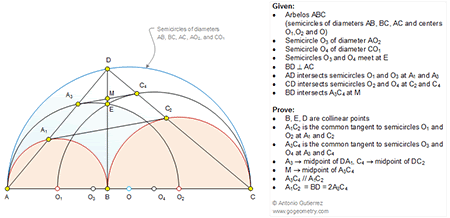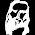## Sunday, December 28, 2014

### Geometry Problem 1071: Arbelos, Semicircles, Diameter, Circle, Perpendicular, Common Tangent, Parallel, Collinear Points, Midpoint, 90 Degrees

Level: Mathematics Education, High School, Honors Geometry, College.

Click the diagram below to enlarge it.#### 1 comment:

1.Let BD and A₁C₂ intersect at P.

Join A₁B and BC₂. Then A₁BC₂D is a rectangle.
Thus PA₁=PB=PC₂=PD, and so A₁C₂ is the common tangent of O₁ and O₂.

***
Clearly, A₁B//A₃O₂//DC, and BO₂=O₂C, so A₁A₃=A₃D.
Similarly, C₂C₄=C₄D. Therefore, A₁C₂//A₃C₄ and A₁C₂=2×A₃C₄.

Since A₁BC₂D is rectangle, thus A₁C₂=BD=2×A₃C₄.
Also since P is mid-point of A₁C₂, so M is mid-point of A₃C₄.

***
Since A₁O₁//A₃B//DO₄, and also A₁C₂//A₃C₄,
so A₃C₄ is tangent to circle O₂.

Similarly A₃C₄ is tangent to circle O₄.
And hence A₃C₄ is the common tangent of O₂ and O₄.

Since BD passes through M, and M is mid-point of A₃C₄,
thus M lies on the radical axis of O₂ and O₄, which is BD.
Therefore BD passes through E, which also lies on the radical axis of O₂ and O₄.
Hence, B,E,D are collinear.